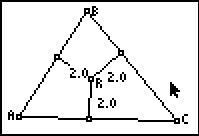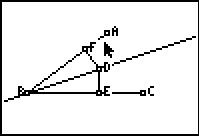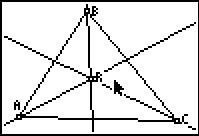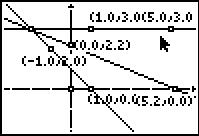# Activities

••• ##### Subject Area

• Math: Geometry: Analytic Geometry
• Math: Geometry: Triangles

• ##### Author9-12

45 Minutes

• ##### Device
• TI-83 Plus Family
• TI-84 Plus
• TI-84 Plus Silver Edition
•TI-84 Plus C Silver Edition
•TI-84 Plus CE
• ##### Software

TI Connect™
TI Connect™ CE

• ##### Accessories

TI Connectivity Cable

## Hanging with the Incenter#### Activity Overview

In this activity, students will explore the perpendicular bisector theorem and discover that if a point is on the perpendicular bisector of a segment, then the point is equidistant from the endpoints.

#### Key Steps

•Students will be exploring the distance from a point on the angle bisector to the segment. They will discover that if a point is on the angle bisector of an angle, then it is equidistant from the two segments of the angle.

•Students are to create an acute triangle and find the angle bisector of all three angles of the triangle. They will also find the distance from the incenter to the three sides of the triangle.

•Problem 3 is an extension of the activity. Students will use the graphing calculator and the angle bisectors to find the coordinates of fence posts on a plot of land.